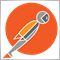# How To Get the Angle Values of TrendLines - OBJPROP_ANGLE21

Hey,

I'm on a script to test something but I have a problem. I have an indicator that draws trendlines on the charts. As you in the pic (attached) I can see it's angle value (343.3) but when I try to call it in the codes by OBJPROP_ANGLE I get 0 value. I tried my best on this but I couldn't solve it. Here is the code that I get 0 value for the "HL_1" object.

I would appreciate all the helps.
```void OnStart()
{
//---
HL1 = iCustom(NULL,0,"Trend Çizgileri",0,1);
LL1 = iCustom(NULL,0,"Trend Çizgileri",1,1);

if(ObjectFind("HL_1")==0 && ObjectFind("LL_1")==0)
{
double anglesLL = ObjectGetDouble(0,"LL_1",OBJPROP_ANGLE);
Alert("Low Trendline Angle: " + anglesLL);
double anglesHL = ObjectGetDouble(0,"HL_1",OBJPROP_ANGLE);
Alert("High Trendline Angle: " + anglesHL);
}```
Files:2744

weyhub:
Hey,

I'm on a script to test something but I have a problem. I have an indicator that draws trendlines on the charts. As you in the pic (attached) I can see it's angle value (343.3) but when I try to call it in the codes by OBJPROP_ANGLE I get 0 value. I tried my best on this but I couldn't solve it. Here is the code that I get 0 value for the "HL_1" object.

I would appreciate all the helps.

You need to use slope (rise/run) instead of angle. Angle changes depending on the zoom of your chart and has no relevance. For example I could have that same chart and move it from my landscape monitor over to my portrait monitor and the angle will be dramatically different. Did the market dynamics change? No, just my monitor. Slope on the other-hand will not change. That is the indicator you are looking for.24244

Can't be done.
1. There is no angle from 2 anchor points. An angle requires distance divided by distance; a unit-less number. A chart has price and time. What is the angle of going 30 miles in 45 minutes? Meaningless!

2. You can get the slope from a trendline: m=ΔPrice÷ΔTime. Changing the price scale, bar width, or window size, changes the apparent angle, the slope is constant. Angle is meaningless.

3. You can create an angled line using one point and setting the angle (Object Properties - Objects Constants - Standard Constants, Enumerations and Structures - MQL4 Reference.) The line is drawn that angle in pixels. Changing the axes scales does NOT change the angle (and thus is meaningless.)

4. If you insist, take the two price/time coordinates, convert them to pixel coordinates and then to apparent angle with arctan.
How to get the angle of a trendline and place a text object to it? - Trend Indicators - MQL4 programming forum - Page 2